Study P1 Mathematics Numbers to 100 - Geniebook# Numbers To 100

## Numbers to 100:

1. Counting to 100
2. Place value

## 1. Counting To 100

Look at the pencil holders and count in tens.Please remember that after 90, we have 100 or One Hundred, not Tenty.

Example:

There are 10 sticks in each bundle. Let’s count on in tens and ones to find the total number of sticks.There are a total of __________ sticks.

65

Explanation: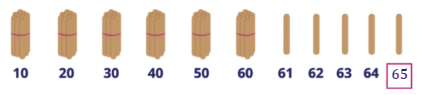Count the bundles in tens. There are 6 bundles, which gives us 60.

Count on from 60:

61, 62, 63, 64, 65

Question 1:

There are 10 sticks in each bundle. Let’s count on in tens and ones to find the total number of sticks.There are a total of __________ sticks.

1. 5
2. 23
3. 30
4. 32

(4) 32

Explanation: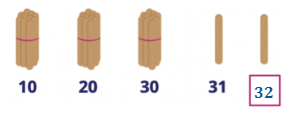Count the bundles in tens. There are 3 bundles, which gives us 30.

Count on from 30:

31, 32

Question 2:

There are 10 sticks in each bundle. Let’s count on in tens to find the total number of sticks.There are a total of __________ sticks.

1. 36
2. 38
3. 48
4. 84

(3) 48

Explanation:Count the bundles in tens. There are 4 bundles, which gives us 40.

Count on from 40:

41, 42, 43, 44, 45, 46, 47, 48

Question 3:

There are 10 sticks in each bundle. Let’s count in tens to find the total number of sticks.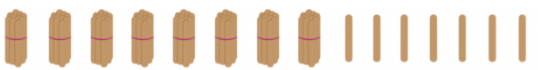There are a total of __________ sticks.

1. 15
2. 78
3. 87
4. 97

(3) 87

Explanation:Count the bundles in tens. There are 8 bundles, which gives us 80.

Count on from 80:

81, 82, 83, 84, 85, 86, 87

Question 4:

There are 10 sticks in each bundle. Let’s count on in tens to find the total number of sticks.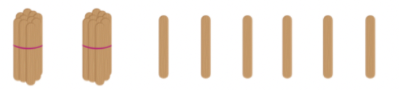There are a total of __________ sticks.

1. Sixteen
2. Twenty-six
3. Twenty-eight
4. Sixty-two

(2) Twenty-six

Explanation:Count the bundles in tens. There are 2 bundles, which gives us 20.

Count on from 20:

21, 22, 23, 24, 25, 26

Question 5:

There are 10 sticks in each bundle. Let’s count on in tens to find the total number of sticks.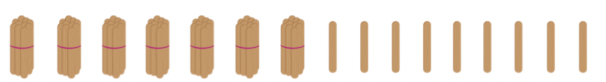There are a total of __________ sticks.

1. Sixty-seven
2. Seventy-eight
3. Seventy-nine
4. Ninety-seven

(3) Seventy-nine

Explanation:Count the bundles in tens. There are 7 bundles, which gives us 70.

Count on from 70:

71, 72, 73, 74, 75, 76, 77, 78, 79

Question 6:

Look at the picture below. How many cars are there?1. 50
2. 59
3. 60
4. 95

(2) 59

Explanation:

There are ten cars in a row. There are five rows of ten, which give us fifty.

There are also 9 cars in the last row.

Therefore, we have 5 tens and 9 ones, which will be 59.

Question 7:

Look at the picture below. How many birds are there?1. Sixty-five
2. Sixty-six
3. Fifty-five
4. Fifty-six

(1) Sixty-five

Explanation:

There are six rows of tens, which give us sixty. There are also five birds in the last row. Therefore, we have 6 tens and 5 ones, which will be 65.

Question 8:

Look at the picture below. How many pandas are there?1. Three
2. Thirteen
3. Thirty
4. Thirty-three

(3) Thirty

Explanation:

There are ten pandas in a row. There are three rows of tens, which give us thirty.

Question 9:

How many lollipops are there in the picture below?1. Twenty-one
2. Twenty-four
3. Twenty-six
4. Twenty-nine

(1) Twenty-one

Question 10:

Match the following:Question 11:

Which of the following shows sixty-two in numeral?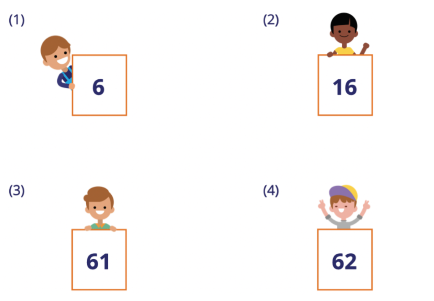(4) 62

Question 12:

Which of the following shows ninety in numeral?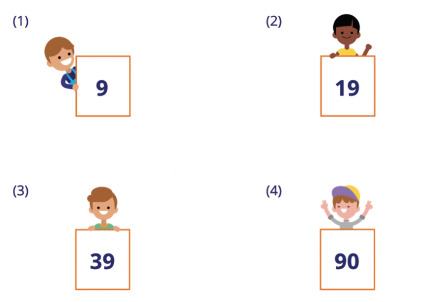(4) 90

Question 13:

Write 52 in words.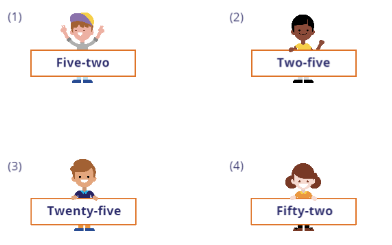(4) Fifty-two

Question 14:

Write 78 in words.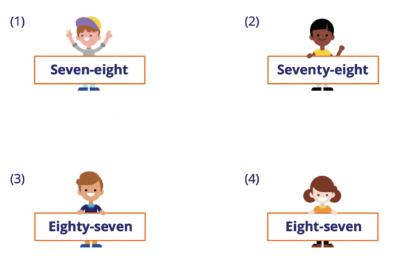(2) Seventy-eight

Question 15:

Write 41 in words.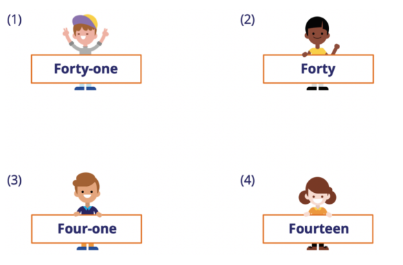(1) Forty-one

## 2. Place Value

Place values tell you the number of tens and ones that we have.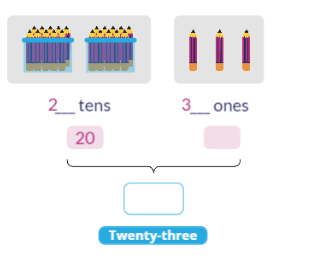\begin{align}​ 23 = 2 \text{ tens } 3 \text{ ones} \\[2ex] 20 \text{ and } 3 \text{ make } 23 \end{align}

Example:

Fill in the blanks and find the total number of pencils.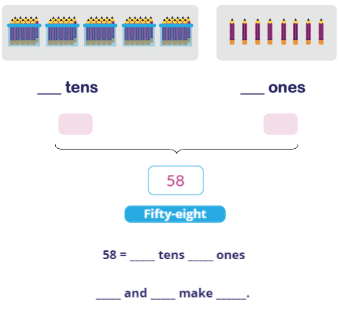58 =  tens and  ones

50  and  make  58 .

Question 1:

Fill in the blanks and find the total number of pencils.__________ tens                                                       __________ ones

99 $$=$$ __________ tens __________ ones

__________ and __________ make __________.

99 $$=$$ 9 tens 9 ones

90 and 9 make 99

Question 2:

How many oranges are there?1. 35
2. 36
3. 45
4. 46

(4) 46

Explanation:

There are 4 tens and 6 ones.

Question 3:

Fill in the blanks:

46 $$=$$ __________ tens __________ ones

1. 4, 6
2. 6, 4
3. 4, 5
4. 5, 4

(1) 4, 6

Question 4:

How many mangoes are there?1. 9
2. 52
3. 72
4. 92

(3) 72

Explanation:

There are 7 tens and 2 ones.

Question 5:

Fill in the blanks:

72 $$=$$ __________ tens __________ ones

1. 1, 7
2. 2, 7
3. 7, 2
4. 7, 1

(3) 7, 2

Question 6:

Count and fill in the blanks: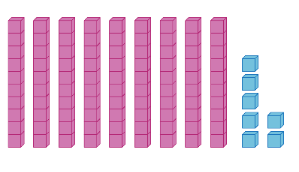__________ $$=$$ __________ tens __________ ones

1. 78 $$=$$ 7 tens 8 ones
2. 79 $$=$$ 7 tens 9 ones
3. 97 $$=$$ 9 tens 7 ones
4. 98 $$=$$ 9 tens 8 ones

(3) 97 $$=$$ 9 tens 7 ones

Explanation:

Each pink stack consists of 10 cubes. There are 9 stacks of ten, which give us 90.

There are also 7 blue cubes.

Therefore, we have 9 tens and 7 ones, which will be 97.

Question 7:

Count and fill in the blanks: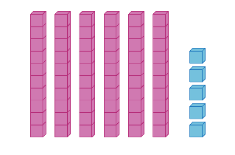__________ $$=$$ __________ tens __________ ones

1. 55 $$=$$ 5 tens 5 ones
2. 56 $$=$$ 5 tens 6 ones
3. 65 $$=$$ 6 tens 5 ones
4. 66 $$=$$ 6 tens 6 ones

(3) 65 $$=$$ 6 tens 5 ones

Explanation:

Each pink stack consists of 10 cubes. There are 6 stacks of tens, which give us 60.

There are also 5 blue cubes.

Therefore, we have 6 tens and 5 ones, which will be 65.

Question 8:

Count and fill in the blanks:__________ $$=$$ __________ ten(s) __________ ones

1. 13 $$=$$ 3 tens 1 ones
2. 13 $$=$$ 1 ten 3 ones
3. 31 $$=$$ 3 tens 1 ones
4. 31 $$=$$ 1 ten 3 ones

(2) 13 $$=$$ 1 ten 3 ones

Question 9:

Count and fill in the blanks:__________ $$=$$ __________ ten(s) __________ ones

1. 26 $$=$$ 2 tens 6 ones
2. 27 $$=$$ 2 tens 7 ones
3. 28 $$=$$ 2 tens 8 ones
4. 72 $$=$$ 7 tens 2 ones

(2) 27 $$=$$ 2 tens 7 ones

Question 10:

Fill in the blank.

2 tens $$=$$ __________

1. 10
2. 2
3. 20
4. 30

(3) 20

Question 11:

Fill in the blank.

5 tens $$=$$ __________

1. 5
2. 10
3. 20
4. 50

(4) 50

Question 12:

What is 1 ten 2 ones?

1. 12
2. 2
3. 3
4. 102

(1) 12

Question 13:

What is 3 tens 5 ones?

1. 8
2. 30
3. 35
4. 53

(3) 35

Question 14:

Fill in the blank.

14 $$=$$  __________ ten 4 ones

1. 1
2. 2
3. 3
4. 4

(1) 1

Question 15:

Fill in the blank.

72 $$=$$ 7 tens __________ ones

1. 1
2. 2
3. 5
4. 7

(2) 2

Question 16:

Fill in the blank:

98 $$=$$ 9 __________ 8 ones

1. Ones
2. Tens
3. Hundreds

(2) Tens

Question 17:

Fill in the blank.

80 and __________ make 81

1. 1
2. 2
3. 3
4. 4

(1) 1

Question 18:

Fill in the blank:

__________ and 4 make 64.

1. 6
2. 40
3. 60
4. 4

(3) 60

Question 19:

Fill in the blank:

39 is __________ and __________.

1. 3, 9
2. 30, 19
3. 3, 19
4. 30, 9

(4) 30, 9

## Challenge Yourself!

Question 1:

Fill in the blank:

100 $$=$$ 1 __________

1. One
2. Ten
3. Hundred

(3) Hundred

## We have learnt the following:

• Numbers to 100
• Counting to 100
• Place value
•   10 $$=$$ 1 ten
•   20 $$=$$ 2 tens
•   36 $$=$$ 3 tens 6 ones
•   59 $$=$$ 5 tens 9 ones
•   75 $$=$$ 7 tens 5 ones
• 100 $$=$$ 1 hundred

Continue Learning
Numbers To 10 Picture Graphs 1
Shapes 1 Ordinal Numbers
Subtraction Within 20 Addition Within 10
Addition And Subtraction Within 10 Numbers To 20
Addition Within 20 Numbers To 100PrimaryPrimary 1EnglishMaths
Numbers To 10
Picture Graphs 1
Shapes 1
Ordinal Numbers
Subtraction Within 20
Numbers To 20
Numbers To 100Primary 2Primary 3Primary 4Primary 5Primary 6SecondarySecondary 1Secondary 2Secondary 3Secondary 4